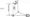# Kinematics of Machines June 2012

Note: 1 .Answer any FIVE full questions, selecting at least TWO questions from each part.

2. Graphical solutions may be obtained on graph sheets or on answer book itself.

PART- A

1 a. Define the following : i) Kinematic chain ii) Mechanism iii) Structure

iv) Inversions v) Degrees of freedom.

b. Sketch and explain the working of an elliptical trammel. Prove that it traces an ellipse.

2 a. Explain with a neat sketch, crank and slotted lever quick return motion mechanism.

b.  Explain with a neat sketch, pantograph mechanism. State its applications.

c.   Explain with a neat sketch, Geneva wheel mechanism.

3    In the toggle mechanism shown in fig.Q3, the slider D is constrained to move on a horizontal path. The crank OA is rotating in the counter clockwise direction at a speed of 180 rpm. The dimensions of the various links are as follows : OA = 180mm , CB = 240mm, AB = 360mm and BD = 540mm. For the given configuration find ,

a.   Velocity of the slider.

b.  Angular velocity of the links AB, CB and BD.

c.   Velocity of rubbing on the pins of diameter of 30mm at A and D.

d.  Torque applied to the crank OA for a force of 2kN at D.4    a. Explain the procedure for velocity and acceleration of the piston in a reciprocating engine mechanism.

b. Explain how by means of Klein’s construction the acceleration of a reciprocating engine is determined.

PART -B

5. The crank of a reciprocating engine is 90mm long, the connecting rod is 360mm long and the crank rotates at 150rpm clockwise. Find the velocity and acceleration of the piston and the angular velocity and angular acceleration of the connecting rod when the angle which the crank makes with inner dead centre is 30°. Solve the problem through complex algebra.

6 a. Derive the expression for length of path of contact, length of arc of a contact and contact ratio for a pair of involute gears in contact.

b.  The following data relate to a pair of involute spur gear in mesh : Module = 6mm ; Number of teeth on wheel = 49 ; Number of teeth on pinion =17 ; Addendum of pinion and gear wheel interms of module = 1 , find the number of pairs of teeth in contact?

7  a. Sketch and explain different types of gear trains.

b.  Figure Q 7 (b), shows an Epicyclic gear train where the arm A the driver and annular gear D is the follower. The wheel D has 112 teeth and B has 48 teeth. B runs freely on pin P and D is separately driven. The arm A runs at 100 rpm and wheel D at 50 rpm in same direction. Find the speed of wheel B and C.Fig.Q 7 (b)

8 a. The following data relate to a cam profile in which the roller follower moves with uniform acceleration and retardation motion during ascent and descent.

Minimum radius of cam = 25mm ;  Roller radius = 8mm ; Lift = 32 mm ; Offset of follower axis = 12mm towards right ; Angle of ascent = 60° ; Angle of descent = 90° ; Angle of dwell between ascent and descent = 45° ; Speed of cam = 200 rpm clockwise.

Draw the profile of the cam.